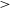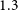Hostname: page-component-797576ffbb-pxgks Total loading time: 0 Render date: 2023-12-02T19:41:29.064Z Has data issue: false Feature Flags: { "corePageComponentGetUserInfoFromSharedSession": true, "coreDisableEcommerce": false, "useRatesEcommerce": true } hasContentIssue false

# WALLABY pilot survey: The diversity of HI structural parameters in nearby galaxies

Published online by Cambridge University Press:  08 June 2023

*
Corresponding author: T. N. Reynolds; Email: tristan.reynolds@uwa.edu.au.

## Abstract

We investigate the diversity in the sizes and average surface densities of the neutral atomic hydrogen (H i) gas discs in$\sim$280 nearby galaxies detected by the Widefield ASKAP L-band Legacy All-sky Blind Survey (WALLABY). We combine the uniformly observed, interferometric H i data from pilot observations of the Hydra cluster and NGC 4636 group fields with photometry measured from ultraviolet, optical, and near-infrared imaging surveys to investigate the interplay between stellar structure, star formation, and H i structural parameters. We quantify the H i structure by the size of the H i relative to the optical disc and the average H i surface density measured using effective and isodensity radii. For galaxies resolved by$>$$1.3$ beams, we find that galaxies with higher stellar masses and stellar surface densities tend to have less extended H i discs and lower H i surface densities: the isodensity H i structural parameters show a weak negative dependence on stellar mass and stellar mass surface density. These trends strengthen when we limit our sample to galaxies resolved by$>$2 beams. We find that galaxies with higher H i surface densities and more extended H i discs tend to be more star forming: the isodensity H i structural parameters have stronger correlations with star formation. Normalising the H i disc size by the optical effective radius (instead of the isophotal radius) produces positive correlations with stellar masses and stellar surface densities and removes the correlations with star formation. This is due to the effective and isodensity H i radii increasing with mass at similar rates while, in the optical, the effective radius increases slower than the isophotal radius. Our results are in qualitative agreement with previous studies and demonstrate that with WALLABY we can begin to bridge the gap between small galaxy samples with high spatial resolution H i data and large, statistical studies using spatially unresolved, single-dish data.

## Keywords

Type
Research Article
© The Author(s), 2023. Published by Cambridge University Press on behalf of the Astronomical Society of Australia

## Access options

Get access to the full version of this content by using one of the access options below. (Log in options will check for institutional or personal access. Content may require purchase if you do not have access.)

## References

Bigiel, F., et al. 2008, AJ, 136, 2846CrossRefGoogle Scholar
Bigiel, F., et al. 2010, AJ, 140, 1194CrossRefGoogle Scholar
Broeils, A. H., & Rhee, M. H. 1997, A&A, 324, 877Google Scholar
Catinella, B., et al. 2018, MNRAS, 476, 875CrossRefGoogle Scholar
Cayatte, V., Kotanyi, C., Balkowski, C., & van Gorkom, J. H. 1994, AJ, 107, 1003CrossRefGoogle Scholar
Chambers, K. C., et al. 2016, arXiv e-prints, arXiv:1612.05560Google Scholar
Chapman, J. M. 2015, in IAU General Assembly, Vol. 29, 2232458Google Scholar
Chen, X., Wang, J., & Kong, X. 2022, ApJ, 933, 39CrossRefGoogle Scholar
Cortese, L., Catinella, B., & Smith, R. 2021, PASA, 38, e035CrossRefGoogle Scholar
Cortese, L., et al. 2012, A&A, 544, A101CrossRefGoogle Scholar
de Vaucouleurs, G. 1948, AnAp, 11, 247Google Scholar
Ferreras, I., Lisker, T., Carollo, C. M., Lilly, S. J., & Mobasher, B. 2005, ApJ, 635, 243CrossRefGoogle Scholar
Flewelling, H. A., et al. 2016, arXiv e-prints, arXiv:1612.05243Google Scholar
Giovanelli, R., et al. 2005, AJ, 130, 2598CrossRefGoogle Scholar
Gunn, J. E., & Gott, III, J. R. 1972, ApJ, 176, 1CrossRefGoogle Scholar
Hall, M., Courteau, S., Dutton, A. A., McDonald, M., & Zhu, Y. 2012, MNRAS, 425, 2741CrossRefGoogle Scholar
Holmberg, E. 1958, Meddelanden fran Lunds Astronomiska Observatorium Serie II, 136, 1Google Scholar
Hotan, A. W., et al. 2021, PASA, 38, e009Google Scholar
Huynh, M., Dempsey, J., Whiting, M. T., & Ophel, M. 2020, in ASP Conference Series, Vol. 522, Astronomical Data Analysis Software and Systems XXVII, ed. P. Ballester, J. Ibsen, M. Solar, & K. Shortridge, Astronomical Society of the Pacific, San Francisco, 263Google Scholar
Janowiecki, S., Catinella, B., Cortese, L., Saintonge, A., & Wang, J. 2020, MNRAS, 493, 1982CrossRefGoogle Scholar
Johnston, S., et al. 2008, ExA, 22, 151CrossRefGoogle Scholar
Jonas, J., & MeerKAT Team. 2016, in MeerKAT Science: On the Pathway to the SKA, 1 Google Scholar
Koribalski, B. S., et al. 2020, Ap SS, 365, 118Google Scholar
Lauberts, A., & Valentijn, E. A. 1989, The Surface Photometry Catalogue of the ESO-Uppsala Galaxies (European Southern Observatory)Google Scholar
Lee, B., et al. 2022, ApJS, 262, 31Google Scholar
Leroy, A. K., et al. 2008, AJ, 136, 2782CrossRefGoogle Scholar
Liller, M. H. 1960, ApJ, 132, 306CrossRefGoogle Scholar
Martin, C. L., & Kennicutt, R. C., Jr. 2001, ApJ, 555, 301CrossRefGoogle Scholar
Martin, D. C., et al. 2005, ApJ, 619, L1Google Scholar
Meurer, G. R., et al. 2018, MNRAS, 476, 1624CrossRefGoogle Scholar
Meyer, M., et al. 2017, PASA, 34, 52Google Scholar
Moore, B., Katz, N., Lake, G., Dressler, A., & Oemler, A. 1996, Natur, 379, 613CrossRefGoogle Scholar
Moore, B., Lake, G., Quinn, T., & Stadel, J. 1999, MNRAS, 304, 465CrossRefGoogle Scholar
Morrissey, P., et al. 2007, ApJS, 173, 682Google Scholar
Muñoz-Mateos, J. C., et al. 2015, ApJS, 219, 3CrossRefGoogle Scholar
Naluminsa, E., Elson, E. C., & Jarrett, T. H. 2021, MNRAS, 502, 5711CrossRefGoogle Scholar
Noordermeer, E., van der Hulst, J. M., Sancisi, R., Swaters, R. A., & van Albada, T. S. 2005, A&A, 442, 137CrossRefGoogle Scholar
Pan, Z., Wang, J., Zheng, X., & Kong, X. 2021, ApJ, 922, 235CrossRefGoogle Scholar
Planck Collaboration, et al. 2016, A&A, 594, A13Google Scholar
Rajohnson, S. H. A., et al. 2022, MNRAS, 512, 2697CrossRefGoogle Scholar
Rasmussen, J., Ponman, T. J., & Mulchaey, J. S. 2006, MNRAS, 370, 453CrossRefGoogle Scholar
Rasmussen, J., et al. 2012, ApJ, 747, 31CrossRefGoogle Scholar
Redman, R. O. 1936, MNRAS, 96, 588CrossRefGoogle Scholar
Reynolds, T. N., et al. 2022, MNRAS, 510, 1716Google Scholar
Saintonge, A., & Catinella, B. 2022, ARA&A, 60, 319CrossRefGoogle Scholar
Saintonge, A., & Spekkens, K. 2011, ApJ, 726, 77CrossRefGoogle Scholar
Sánchez Almeida, J. 2020, MNRAS, 495, 78Google Scholar
Schmidt, T. M., Bigiel, F., Klessen, R. S., & de Blok, W. J. G. 2016, MNRAS, 457, 2642CrossRefGoogle Scholar
Stevens, A. R. H., et al. 2019, MNRAS, 490, 96Google Scholar
Swaters, R. A., van Albada, T. S., van der Hulst, J. M., & Sancisi, R. 2002, A&A, 390, 829CrossRefGoogle Scholar
Toomre, A., & Toomre, J. 1972, ApJ, 178, 623CrossRefGoogle Scholar
Trujillo, I., Chamba, N., & Knapen, J. H. 2020, MNRAS, 493, 87CrossRefGoogle Scholar
Trujillo, I., Graham, A. W., & Caon, N. 2001, MNRAS, 326, 869CrossRefGoogle Scholar
Verheijen, M. A. W., et al. 2008, in American Institute of Physics Conference Series, Vol. 1035, The Evolution of Galaxies Through the Neutral Hydrogen Window, ed. Minchin, R., & Momjian, E., 265–271Google Scholar
Walter, F., et al. 2008, AJ, 136, 2563CrossRefGoogle Scholar
Wang, J., et al. 2013, MNRAS, 433, 270Google Scholar
Wang, J., et al. 2014, MNRAS, 441, 2159Google Scholar
Wang, J., et al. 2016, MNRAS, 460, 2143Google Scholar
Wang, J., et al. 2017, MNRAS, 472, 3029CrossRefGoogle Scholar
Wang, J., et al. 2020, ApJ, 890, 63CrossRefGoogle Scholar
Wang, J., et al. 2021, ApJ, 915, 70CrossRefGoogle Scholar
Westmeier, T., Braun, R., & Koribalski, B. S. 2011, MNRAS, 410, 2217CrossRefGoogle Scholar
Westmeier, T., Deg, N., Spekkens, K., Reynolds, T., & Shen, A. 2022, PASAGoogle Scholar
Westmeier, T., et al. 2021, MNRAS, 506, 3962CrossRefGoogle Scholar
Wright, E. L., et al. 2010, AJ, 140, 1868Google Scholar